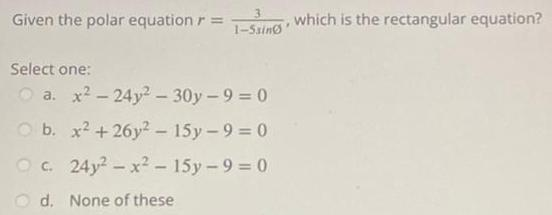Question:

# Given the polar equation r 1 5sing Select one O a x 24y 30y

Last updated: 7/9/2023Given the polar equation r 1 5sing Select one O a x 24y 30y 9 0 O b x 26y2 15y 9 0 Oc 24y x 15y 9 0 d None of these which is the rectangular equation